# Boats and Stream Questions

Boats and Stream Questions in English for the SSC CGL, CPO, CHSL, Bank and all competitive exams. Practice set of previous year MCQ question for the preparation of upcoming examinations.

## Boats and Stream Questions Practice Set

Question 1: A man rows a boat 18 kilometres in 4 hours downstream and returns upstream in 12 hours. The speed of the stream (in km per hour) is :
(1) 1
(2) 1.5
(3) 2
(4) 1.75

(2) 1.5
Rate downstream=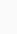$\dfrac{18}{4}$=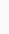$\dfrac{9}{2}$=4.5 km/hr
Rate upstream =$\dfrac{18}{12}$ = 1.5 km/hr
speed of the stream=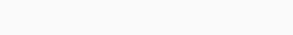$\dfrac{speed of downstream - speed of upstream}{2}$ =$\dfrac{3}{2}$= 1.5 km/hr

Question 2: A motorboat in still water travels at a speed of 36 kmph. It goes 56 km upstream in 1 hour 45 minutes. The time taken by it to cover the same distance down the stream will be :
(1) 2 hours 25 minutes
(2) 3 hours
(3) 1 hour 24 minutes
(4) 2 hours 21 minutes

(3) 1 hour 24 minutes
Speed of the motorboat upstream=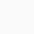$\dfrac{56x4}{7}$ = 32 kmph
Let the speed of the current be x kmph=36 – x = 32, x= 4 kmph
Speed of motor boat downstream = 36 + 4 = 40 kmph
Time taken to cover 56 km at 40 kmph =$\dfrac{56}{40}$
=$\dfrac{7}{5}$ hour= 1 hour 24 minutes

Question 3: The speed of a boat in still water is 10 km/hr. It covers (upstream) a distance of 45 km in 6 hours. The speed (in km/hr) of the stream is
(1) 2.5
(2) 3
(3) 3.5
(4) 4

(1) 2.5
Upstream speed of boat=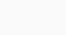$\dfrac{Distance}{Time}$
=$\dfrac{45}{6}$=7.5 km/hr
Speed of current = 10-7.5=2.5 km/hr

Question 4: A man rows 40 km upstream in 8 hours and a distance of 36 km downstream in 6 hours. Then speed of stream is
(1) 0.5 km/hr
(2) 1.5 km/hr
(3) 1 km/hr
(4) 3 km/hr

(1) 0.5 km/hr
Speed of stream=$\dfrac{1}{2}$ (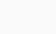$\dfrac{36}{6} - \dfrac{40}{8}$)
=$\dfrac{1}{2}$= 0.5 km/hr

Question 5: A boat travels 24 km upstream in 6 hours and 20 km downstream in 4 hours. Then the speed of boat in still water and the speed of water current are respectively
(1) 4 kmph and 3 kmph
(2) 4.5 kmph and 0.5 kmph
(3) 4 kmph and 2 kmph
(4) 5 kmph and 2 kmph

(2) 4.5 kmph and 0.5 kmph
Rate upstream = 4 kmph
Rate downstream = 5 kmph
Speed of boat in still water=$\dfrac{1}{2}$ (4+5)=4.5 kmph
Speed of current=$\dfrac{1}{2}$ (5-4)=0.5 kmph

Question 6: If a boat goes 100 km downstream in 10 hours and 75 km upstream in 15 hours, then the speed of the stream is
(1) 2 km/hour
(2) 2·5 km/hour
(3) 3 km/hour
(4) 3·5 km/hour

(2) 2·5 km/hour
Rate downstream = 10 kmph
Rate upstream = 5 kmph
Speed of current=$\dfrac{1}{2}$ (10-5)
=2·5 km/hour

Question 7: A man can swim at the rate of 4 km/hr in still water. If the speed of the water is 2 km/hr, then the time taken by him to swim 10 km upstream is
(1) 2$\frac{1}{2}$hrs
(2) 3$\frac{1}{2}$hrs
(3) 5 hrs
(4) 4 hrs

Rate upstream = 4 – 2 = 2 kmph
Required time =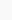$\frac{10}{2}$= 5 hrs

Question 8: A man can swim 3 km/hr. in still water. If the velocity of the stream is 2 km/hr., the time taken by him to swim to a place 10 km upstream and back is :
(1) 9$\frac{1}{3}$ hr
(2) 10 hr
(3) 12 hr
(4) 8$\frac{1}{3}$ hr

(3) 12 hr
Rate downstream = 5 kmph
Rate upstream = 1 kmph
Required time=$\dfrac{10}{5}$ +$\dfrac{10}{1}$
=12 hours

Question 9: A man can row 15km/ hr downstream and 9 km/hr upstream. The speed of the boat in still water is
(1) 8 km/hr
(2) 10 km/hr.
(3) 15 km/hr
(4) 12 km/ hr

Speed of boat in still water
=$\dfrac{1}{2}$(Rate downstream + Rate upstream) =
=$\dfrac{1}{2}$(15 + 9)
= 12 kmph

Question 10: A man swims downstream a distance of 15 km in 1 hour. If the speed of the current is 5 km/hour, the time taken by the man to swim the same distance upstream is
(1) 1 hour 30 minutes
(2) 45 minutes
(3) 2 hours 30 minutes
(4) 3 hours

(4) 3 hours
Let the speed of man in still water be x kmph.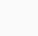$\dfrac{15}{5+x}$ =1
x + 5 = 15
x = 10 kmph
Time taken in swimming upstream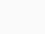$\dfrac{15}{10-5}$ = 3 hour

Question 11: A man rows down a river 15 km in 3 hrs. with the stream and returns in 7$\dfrac{1}{2}$ hrs, The rate at which he rows in still water is
(1) 2.5 km/hr
(2) 1.5 km/hr
(3) 3.5 km/hr
(4) 4.5 km/h

(3) (3) 3.5 km/hr
Let speed of person in still water = x kmph and speed of current = y kmph
x+y =$\dfrac{15}{3}$ = 5 kmph
x-y=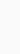$\dfrac{15}{ \dfrac{15}{2}}$= 2 kmph
2x= 7 kmph
x=3.5 kmph

Question 12 : A boat takes half time in moving a certain distance downstream than upstream. The ratio of the speed of the boat in still water and that of the current is
(1) 2 : 1
(2) 1 : 2
(3) 4 : 3
(4) 3 : 1

(4) 3 : 1
Speed of boat in still water = x kmph (let)
Speed of current = y kmph
Rate downstream = (x + y) kmph
Rate upstream = (x – y) kmph
Distance = Speed × Time
(x – y) × 2t = (x + y) × t
2x – 2y = x + y
2x – x = 2y + y
x = 3y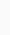$\dfrac{x}{y}$ =$\dfrac{3}{1}$ = 3 : 1

Question 13: A boat moves downstream at the rate of 8 km per hour and upstream at 4 km per hour. The speed of the boat in still waters is :
(1) 4.5 km per hour
(2) 5 km per hour
(3) 6 km per hour
(4) 6.4 km per hour

(3) 6 km per hour
Speed of boat in still water
=$\dfrac{1}{2}$ (Rate downstream + rate upstream)
=$\dfrac{1}{2}$ (8 + 4) kmph =
=$\dfrac{12}{2}$ kmph
= 6 kmph

Question 14 : A boat goes 6 km an hour in still water, but takes thrice as much time in going the same distance against the current. The speed of the current (in km/hour) is :
(1) 4
(2) 5
(3) 3
(4) 2

(1) 4
Let the speed of the current be x kmph.
According to the question,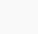$\dfrac{6}{6-x}$= 3
18 – 3x = 6
3x = 18 – 6
x=4

Question 15 : A boat can travel with a speed of 13 km/hr in still water. If the speed of stream is 4 km/hr in the same direction, time taken by boat to go 63 km in opposite direction is
(1) 9 hrs
(2) 4 hrs
(3) 7 hrs
(4) 3$\dfrac{9}{17}$ hrs

(3) 7 hrs
Rate upstream of boat
= 13 – 4 = 9 kmph
Required time =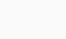$\dfrac{Distance}{speed}$
=$\dfrac{63}{9}$
= 7 hours

Question 16 : A boat covers 20 km in 4 hours along the current and 9 km in 3 hours against the current. What is the speed of the current ?
(1) 2 kmph
(2) 1 kmph
(3) 1.5 kmph
(4) 1.75 kmph

Rate downstream=$\dfrac{20}{4}$ = 5 kmph
Rate upstream =$\dfrac{9}{3}$ = 3 kmph
Speed of current=$\dfrac{1}{2}$(5-3)= 1 kmph

Question 17: A boat running down stream covers a distance of 16 km in 2 hours while for covering the same distance upstream it takes 4 hours. What is the speed of the boat in still water ?
(1) 4 kmph
(2) 6 kmph
(3) 8 kmph

Rate upstream =$\dfrac{16}{2}$ kmph = 8 kmph
Rate downs stream =$\dfrac{16}{4}$= 4 kmph
Rate in still water=$\dfrac{1}{2}$(8+4) kmph = 6 kmph